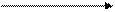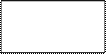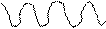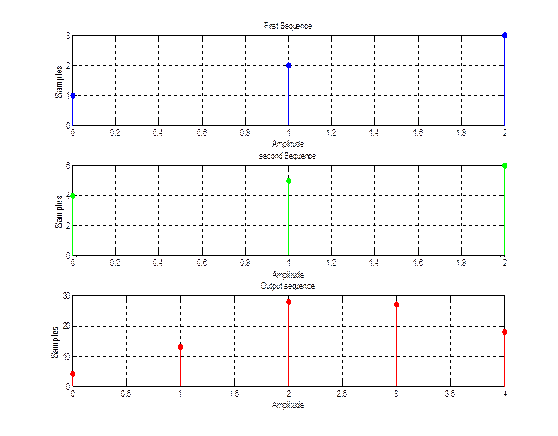EXPERIMENT NO.2

TITLE: FREQUENCY RESPONSE OF LINEAR TIME INVARIANT SYSYTEM

THEORY:

Introduction to LTI system:

Fourier theory is predicated upon LTI (Linear Time Invariant) system. Thus before we even discuss signal processing, it is important to first understand what makes particular system LTI. In this experiment you will learn what classifies a system as LTI and why it plays a fundamental role in Fourier analysis.

There are three requirements of LTI systems:

1. Scalability

3. Time variant

We will see how each property affects following block diagram:Input signal r[n] Output signal y[n]1. Scalability: Scalability means a change in input signalâ€™s amplitude results in corresponding change in output signalâ€™s amplitude. Letâ€™s look at quick example.

In image you can see following input signal produce following output when it is introduced to LTI system. The input is simply shifted to right by two sample points and input amplitude is multiplied by a factor of two. Now letâ€™s multiply input signal by a factor of two and run through LTI system. According to rule of scalability, output would also increase by a factor of two. You can see it illustrated.

2. Additivity: For this example, we use same LTI system as above where input is simply shifted to right by two sample points and amplitude is multiplied by factor of two. If you take a look at the series of images, you will understand basic idea of additivity. The important thing to realize here is that added signals pass through system without interacting. In other words signals added at input produce signals that are added at output. The following image provides an example of additively.

3. Time Invariant: Being time invariant means that the characteristics of system are not dependent on time. This means that shift in input signal will create an identical shift in output signal. For example if my system reacts in a particular way on day 1. It will act in an identical manner 500 days from now! Thus if I have an LTI system which produces following output signal.

SPECIAL PROPERTY OF LTI SYSTEM: One of special property of LTI system in sinusoidal fidelity. What exactly does it mean? It means that if you input sinusoidal into LTI system, then you will get a sinusoidal output. Sinusoidal have special property wherein any sinusoidal input to linear time invariant system results in sinusoidal output that differs in phase shift while returning frequency and wave shape. This is integral to Fourier analysis.LTILThen we deal with LTI system. We can breakdown a very complicated signal to something that is more manageable. This is possible because LTI system have property of being additive and scalable, superposition and decomposition and essentially two different sides to the same thing.

For instance, letâ€™s say we have very complicated signal. How can we go about analyzing it?

i. First we break that signal down into a series of simpler waveforms that are easy to analyze.

ii. Next, we analyze these signals individually.

iii. Finally we sum up the outputs of each individual signals. This gives us output of original complex signal.

This is essentially what is done in Fourier analysis.

MATLAB CODE

Frequency Response of LTI Systems

Xn=input('Enter first sequence X(n)=');

hn=input('Enter second sequence h(n)=');

Yn=conv(hn,Xn);

disp('Yn');

disp(Yn);

xlen=length(Xn)-1;

ylen=length(Yn)-1;

hlen=length(hn)-1;

n=0:1:xlen;

subplot(3,1,1);

stem(n,Xn,'fill','b');

grid on;

xlabel('Amplitude');

ylabel('Samples');

title('First Sequence');

n=0:1:hlen;

subplot(3,1,2);

stem(n,hn,'fill','g');

grid on;

xlabel('Amplitude');

ylabel('Samples');

title('second Sequence');

n=0:1:ylen;

subplot(3,1,3);

stem(n,Yn,'fill','r');

grid on;

xlabel('Amplitude');

ylabel('Samples');

title('Output sequence');

OUTPUT

Enter first sequence X(n)=[1 2 3]

Enter second sequence h(n)=[4 5 6]

Yn

4 13 28 27 18CONCLUSION: